Rotational Kinematics

Key Equationslinear speed v is equal to the angular speed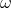multiplied by the radius of the rotating object.linear acceleration a is equal to the angular accelerationmultiplied by the radius of the rotating object.Rotational equivalent of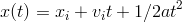Rotational equivalent of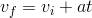Rotational equivalent ofGuidance

These equations work in the case of constant angular acceleration. Use them just as you would use the linear kinematic equations studied in the One-Dimensional Motion lessons. Just replace displacement with the change in angle, the velocity with the angular velocity and the acceleration with the angular acceleration.

• When something rotates in a circle, it moves through a position angle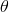that runs from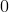toradians and starts over again at. The physical distance it moves is called the path length. If the radius of the circle is larger, the path length traveled is longer.
• The angular velocitytells you how quickly the anglechanges. In more formal language, the rate of change of, the angular position, is called the angular velocity. The direction of angular velocity is either clockwise or counterclockwise. Analogously, the rate of change ofis the angular acceleration.
• The linear velocity and linear acceleration of rotating object also depend on the radius of rotation, which is called the moment arm (See figure below.) If something is rotating at a constant angular velocity, it moves more quickly if it is farther from the center of rotation. For instance, people at the Earth’s equator are moving faster than people at northern latitudes, even though their day is still 24 hours long – this is because they have a greater circumference to travel in the same amount of time.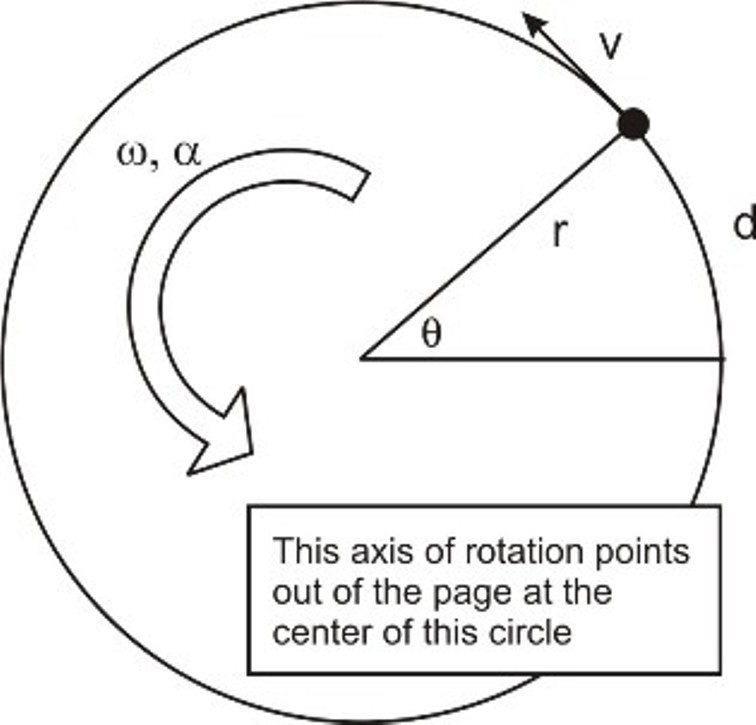#### Example 1

A 2 kg mass is attached to a .5 m long string. Starting from rest, the mass is given a constant angular acceleration of 2 rad/s2. If the string breaks when the tension exceeds 50 N, how long will it be before the string breaks and what will the object's angular displacement be?

##### Solution

To start, we first want to find the object's linear speed when the string breaks.Now, we can find the angular speed.Now we can begin finding the values asked in the problem based on this information using the equations from the section above. We'll start by finding the time before the string breaks.Lastly, we'll find the angular displacement of the mass.